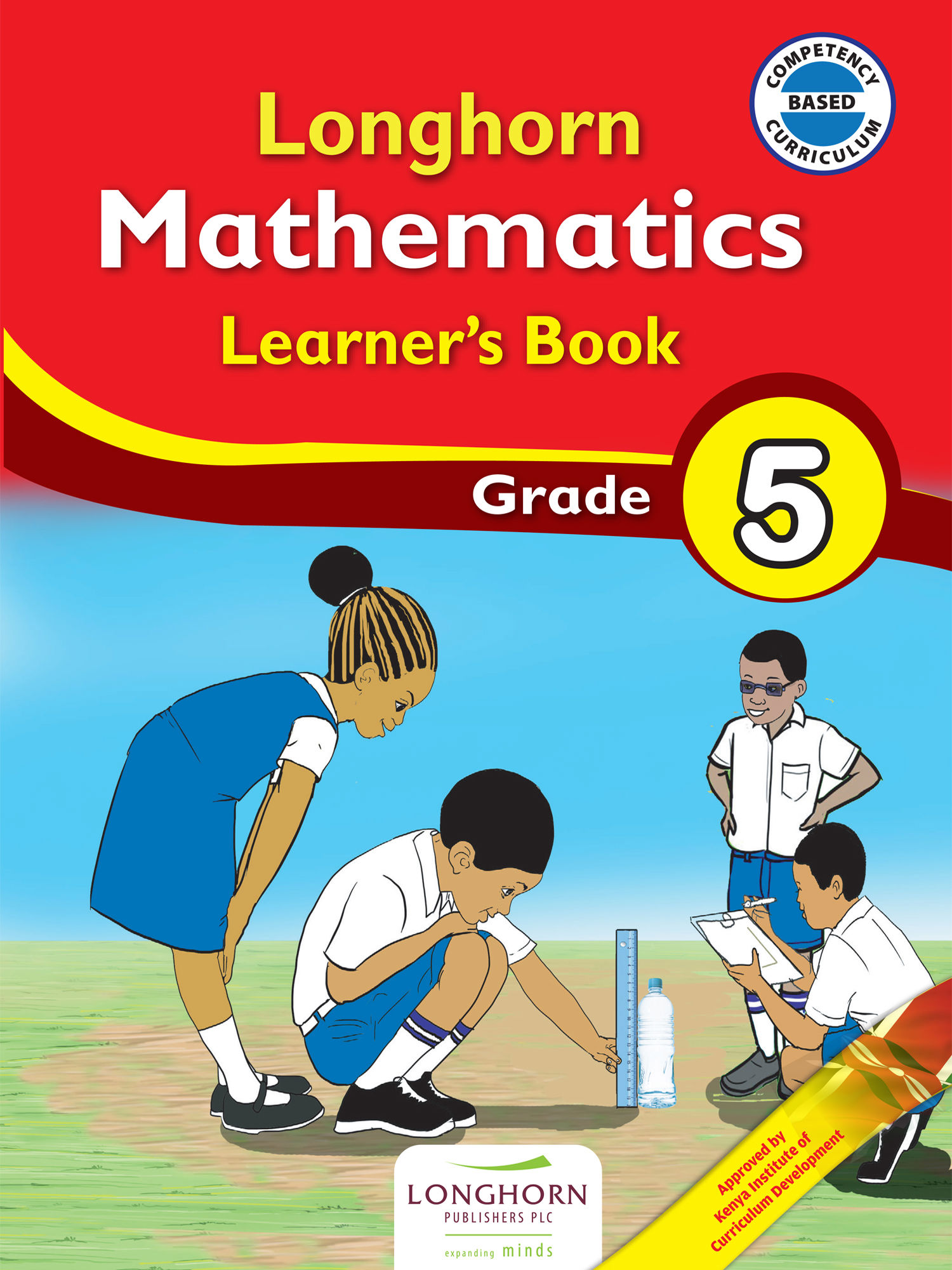Study kit (Mathematics 5th)

# Mathematics 5##### Authors
Tabither Opul, Isaac Ochoo, Thaddeus Mukhuri
##### Exercises kit’s authors
Tabither Opul, Isaac Ochoo, Thaddeus Mukhuri
##### Publisher
Longhorn Publishers
##### Included in packages• The study kit contains 97 chapters and teacher’s exercises kit contains 103 exercises.
• ##### Authors
Tabither Opul, Isaac Ochoo, Thaddeus Mukhuri
• ##### Exercises kit’s authors
Tabither Opul, Isaac Ochoo, Thaddeus Mukhuri
Mathematics
English
• ##### Publisher
Longhorn Publishers

### 1. Numbers – Whole Numbers

##### Chapter
1.1. Place value of digits up to hundreds of thousands
Free chapter!
1.2. Total value of digits up to hundreds of thousands
1.3. Using numbers in symbols
1.4. Reading and writing numbers in symbols
1.5. Reading and writing numbers in words
1.6. Ordering numbers
1.7. Rounding off numbers
1.8. Divisibility of numbers
1.9. Greatest Common Divisor (GCD)
1.10. Least Common Multiple (LCM)

### 3. Numbers – Subtraction

##### Chapter
3.1. Subtraction without regrouping
3.2. Subtraction with regrouping
3.3. Combined operations involving addition and subtraction
3.4. Number patterns involving subtraction

### 4. Numbers – Multiplication

##### Chapter
4.1. Multiplication of a 3-digit number by a 1-digit number
4.2. Multiplication of a 3-digit number by a 2-digit number
4.3. Estimating products of numbers
4.4. Number patterns involving multiplication

### 5. Numbers – Division

##### Chapter
5.1. Dividing up to a 3-digit number by a 1-digit number
5.2. Dividing up to a 3-digit number by a 2-digit number
5.3. Relationship between multiplication and division
5.4. Estimating division by rounding off
5.5. Combined operation

### 6. Numbers – Fractions

##### Chapter
6.1. Equivalent fractions
6.2. Simplifying fractions
6.3. Comparing fractions
6.4. Ordering fractions
6.5. Addition of fractions with same denominator
6.6. Subtraction of fractions with the same denominator
6.7. Addition of fractions with one renaming
6.8. Subtraction of fractions with one renaming

### 7. Numbers – Decimals

##### Chapter
7.1. Introduction to thousandths
7.2. Place value of decimals
7.3. Ordering decimals
7.5. Subtraction involving decimals

### 8. Measurement – Length

##### Chapter
8.1. Kilometre as a unit of measuring length
8.2. Estimating and measuring length in kilometres
8.3. Relationship between kilometre and metre
8.4. Conversion of length
8.5. Addition involving metres and kilometres
8.6. Subtraction involving metres and kilometres
8.7. Multiplication of metres and kilometres by whole numbers
8.8. Division of metres and kilometres by a whole number

### 9. Measurement - Area

##### Chapter
9.1. Units of Area
9.2. Area in square centimetres

### 10. Measurement - Volume

##### Chapter
10.1. Cubic centimetre as a unit of measuring volume
10.2. Volume of cubes and cuboids
10.3. Deriving formula for volume of cubes and cuboids
10.4. Volume of cubes and cuboids using a formula

### 11. Measurement - Capacity

##### Chapter
11.1. Millilitres as a unit of measurement
11.2. Measuring capacity in millilitres
11.3. Measuring capacity in multiples of 5 millilitres
11.4. Relationship between litres and millilitres
11.5. Converting litres to millilitres and millilitres to litres
11.6. Addition involving litres and millilitres
11.7. Subtraction involving litres and millilitres
11.8. Multiplication of litres and millilitres by a whole number
11.9. Division of litres and millilitres by a whole number

### 12. Measurement - Mass

##### Chapter
12.1. Grammes (g) as a unit of measuring mass
12.2. Measuring mass in grammes
12.3. Estimating and measuring mass in grammes
12.4. Relationship between kilogrammes and grammes
12.5. Changing kilogrammes to grammes and grammes to kilogrammes
12.6. Addition involving kilogrammes and grammes
12.7. Subtraction involving kilogrammes and grammes
12.8. Multiplication involving kilogrammes and grammes
12.9. Division involving kilogrammes and grammes

### 13. Measurement - Time

##### Chapter
13.1. Seconds as a unit of measuring time
13.2. Relationship between minutes and seconds
13.3. Addition involving minutes and seconds
13.4. Subtraction involving minutes and seconds
13.5. Multiplication involving time in minutes and seconds by a whole number
13.6. Division involving time in minutes and seconds

### 14. Measurement - Money

##### Chapter
14.1. Budgeting
14.2. Tax
14.3. Services provided by banks
14.4. Saving money

### 15. Geometry - Lines

##### Chapter
15.1. Identifying horizontal and vertical lines
15.2. Drawing horizontal and vertical lines
15.3. Perpendicular lines
15.4. Parallel lines

### 16. Geometry - Angles

##### Chapter
16.1. Relating turns to angles
16.2. Relating turns to angles (2)

### 17. Geometry - 3-D Objects

##### Chapter
17.1. Describing 3-D objects in the environment
17.2. Describing 2-D shapes in 3-D objects

### 18. Data Handling - Data Representation

##### Chapter
18.1. Collecting and representing data using tables
18.2. Representing data by piling objects
18.3. Interpreting data represented by piles